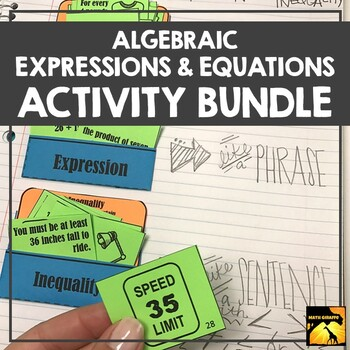# Expressions & Equations Bundle7th - 8th
Subjects
Standards
Resource Type
Formats Included
• Zip
Pages
N/A
\$78.25
List Price:
\$98.00
You Save:
\$19.75
\$78.25
List Price:
\$98.00
You Save:
\$19.75

### Description

This set of activities is perfect for 7th or 8th grade Pre-Algebra, and also offers a great mix of review for Algebra 1 students.

The blend of games, inquiry activities, practice sets, note pages, and puzzles offer a mix of fun and rigor that can be spread throughout the year as supplemental activities!

Check out the preview file for more detail.

Here's what's included:

Order of Operations Doodle Notes

Combining Like Terms Doodle Notes

Combining Like Terms Puzzles

Distributive Property Investigation - Bracelets

Simplifying & Evaluating Expressions - GridWords

Equation Ornaments: Solve and Color

Laws of Exponents Bundle

Order of Operations Conquest Game (no negatives)

Distributive Property Doodle Notes

Evaluating Expressions - Punch Pattern Activity

Thanksgiving Equations

One Step Equations Conquest Game (no negatives)

Two Step Equations Conquest Game (no negatives)

Discovering Laws of Exponents: Inquiry Activity

Writing Equations - "Pass It On" Activity

Exponent Rules War Game

Problem Solving: Writing an Equation Doodle Note

Solving One Step Equations - Conquest Game

Solving Two Step Equations - Conquest Game

Equations With Variables on Both Sides - Conquest Game

Solving Multi-Step Equations Reference Guide

Christmas Equations

Valentine Equations

St. Patrick's Day Equations

Properties of Numbers Doodle Notes

Expressions & Equations - Always, Sometimes, Never

1 Step Equations Doodle Notes

2 Step Equations Doodle Notes

Expression, Equation, or Inequality Card Sort

Variables & Expressions Doodle Notes

Equations & Solutions Doodle Notes

Easter Equations

Total Pages
N/A
Included
Teaching Duration
N/A
Report this Resource to TpT
Reported resources will be reviewed by our team. Report this resource to let us know if this resource violates TpT’s content guidelines.

### Standards

to see state-specific standards (only available in the US).
Write, read, and evaluate expressions in which letters stand for numbers.
Identify parts of an expression using mathematical terms (sum, term, product, factor, quotient, coefficient); view one or more parts of an expression as a single entity. For example, describe the expression 2 (8 + 7) as a product of two factors; view (8 + 7) as both a single entity and a sum of two terms.
Evaluate expressions at specific values of their variables. Include expressions that arise from formulas used in real-world problems. Perform arithmetic operations, including those involving whole-number exponents, in the conventional order when there are no parentheses to specify a particular order (Order of Operations). For example, use the formulas V = s³ and A = 6 s² to find the volume and surface area of a cube with sides of length s = 1/2.
Apply the properties of operations to generate equivalent expressions. For example, apply the distributive property to the expression 3 (2 + 𝘹) to produce the equivalent expression 6 + 3𝘹; apply the distributive property to the expression 24𝘹 + 18𝘺 to produce the equivalent expression 6 (4𝘹 + 3𝘺); apply properties of operations to 𝘺 + 𝘺 + 𝘺 to produce the equivalent expression 3𝘺.
Understand solving an equation or inequality as a process of answering a question: which values from a specified set, if any, make the equation or inequality true? Use substitution to determine whether a given number in a specified set makes an equation or inequality true.